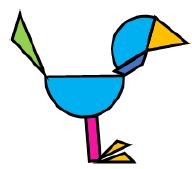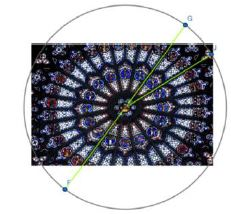# MA.912.GR.6.4Export Print
Solve mathematical and real-world problems involving the arc length and area of a sector in a given circle.

### Clarifications

Clarification 1: Instruction focuses on the conceptual understanding that for a given angle measure the length of the intercepted arc is proportional to the radius, and for a given radius the length of the intercepted arc is proportional is the angle measure.
General Information
Subject Area: Mathematics (B.E.S.T.)
Strand: Geometric Reasoning
Status: State Board Approved

## Benchmark Instructional Guide

• Circle

### Vertical Alignment

Previous Benchmarks

Next Benchmarks

### Purpose and Instructional Strategies

In grade 7, students solved problems involving the circumference and area of a circle. In Algebra 1, students rearranged formulas to highlight a quantity of interest and solved linear equations in one variable. In Geometry, students use their knowledge of circumference and area to relate arc measure to arc length and to area of sectors of circles. In later courses, students will determine the value of trigonometric functions for real numbers by identifying angle measures in the unit circle, and will convert between radians and degrees.
• Instruction includes the student understanding that arcs can be measured in both degrees (arc  measure) and in units of length (arc length). For expectations of Geometry, students will only need to work in degrees when discussing arc measure as students will work with radians in later              courses.
• Instruction includes the understanding that two or more circles with a common center, called concentric circles, will have the same arc measure but different corresponding arc lengths.
• For example, given various concentric circles, students can draw a central angle and extend its side to the length of the radius of the largest circle. Students should notice that the measure of the central angle remains the same and that the larger the circle; the longer the intercepted arc; and that the length of the intercept arc depends proportionally on the radius in the same way that the circumference depends proportionally on the radius.
• When determining an arc length or an area of a sector given the arc measure, instruction includes the connection to proportional relationships (as was done in grade 7).
•  For example, if the arc measure is 57°, and students are asked to find the area of the sector, they can determine the area of the entire circle and multiply by $\frac{\text{57}}{\text{360}}$. Students should realize that areas of sectors are fractional portions of the area of the entire circle.
• For example, if the arc measure is 57°, and students are asked to find the arc length, they can determine the circumference of the entire circle and multiply by $\frac{\text{57}}{\text{360}}$. Students should realize that arc lengths are fractional portions of the circumference of the entire circle

### Common Misconceptions or Errors

• Students may confuse arc measure and arc length, and may try to measure arcs with linear units rather than degrees.

• De’Veon must create an animal using geometric shapes for his Geometry class. He has decided to use construction paper scraps from his mom’s crafting box to create a bird, like the one shown below. The head is a made from a sector with radius 1.5 centimeters and central angle measuring 130°. The body is a semicircle with radius 1.9 centimeters.• Part A. What fraction of the whole circle is the head?
• Part B. How much glitter string will he need to outline the part of the bird’s head that is not touching the beak or neck?
• Part C. What is the total area of light blue construction paper used to create the bird (i.e., the area of the head and the body)?

### Instructional Items

Instructional Item 1
• The North Rose Window in the Rouen Cathedral in France has a diameter of 23 feet. The stained glass design is equally spaced about the center of the circle. What is the area of the sector bounded by arc GJ?*The strategies, tasks and items included in the B1G-M are examples and should not be considered comprehensive.

## Related Courses

This benchmark is part of these courses.
1200400: Foundational Skills in Mathematics 9-12 (Specifically in versions: 2014 - 2015, 2015 - 2022, 2022 and beyond (current))
1206310: Geometry (Specifically in versions: 2014 - 2015, 2015 - 2022, 2022 and beyond (current))
1206320: Geometry Honors (Specifically in versions: 2014 - 2015, 2015 - 2022, 2022 and beyond (current))
1206315: Geometry for Credit Recovery (Specifically in versions: 2014 - 2015, 2015 - 2022, 2022 and beyond (current))
7912065: Access Geometry (Specifically in versions: 2015 - 2022, 2022 and beyond (current))

## Related Access Points

Alternate version of this benchmark for students with significant cognitive disabilities.
MA.912.GR.6.AP.4: Identify and describe the relationship involving the arc length and area of a sector in a given circle.

## Related Resources

Vetted resources educators can use to teach the concepts and skills in this benchmark.

## Formative Assessments

Softball Complex:

Students are asked to solve a design problem in which a softball complex is to be located on a given tract of land subject to a set of specifications.

Type: Formative Assessment

Deriving the Sector Area Formula:

Students are asked to write a formula to find the area of a sector of a circle and then explain and justify that formula.

Type: Formative Assessment

Students are asked to explain why the length of an arc intercepted by an angle is proportional to the radius and then explain how that proportionality leads to a definition of the radian measure of an angle.

Type: Formative Assessment

Sector Area:

Students are asked to find the areas of sectors in two different circles.

Type: Formative Assessment

## Lesson Plans

The Grass is Always Greener:

The lesson introduces area of sectors of circles then uses the areas of circles and sectors to approximate area of 2-D figures. The lesson culminates in using the area of circles and sectors of circles as spray patterns in the design of a sprinkler system between a house and the perimeter of the yard (2-D figure).

Type: Lesson Plan

In this lesson, students will convert from degrees to radians and radians to degrees and calculate arc length using both degrees and radians. Students will come to consensus as to why radians are the preferred measure of an angle. This lesson normally takes two 50 minute class periods to teach.

• Day 1: Bell Ringer-Day 1, Lesson Notes, Activity 1
• Day 2: Day 1 Review and Wrap-up, Bell Ringer-Day 2, Activity 2

Type: Lesson Plan

## Text Resource

Why Tau Trumps Pi:

This informational text resource is intended to support reading in the content area. The author tries to convince the reader that two pi, or tau, occurs more often in mathematics than pi by itself. The author provides several examples and indicates the history behind society's choice of pi rather than tau.

Type: Text Resource

## MFAS Formative Assessments

Students are asked to explain why the length of an arc intercepted by an angle is proportional to the radius and then explain how that proportionality leads to a definition of the radian measure of an angle.

Deriving the Sector Area Formula:

Students are asked to write a formula to find the area of a sector of a circle and then explain and justify that formula.

Sector Area:

Students are asked to find the areas of sectors in two different circles.

Softball Complex:

Students are asked to solve a design problem in which a softball complex is to be located on a given tract of land subject to a set of specifications.

## Student Resources

Vetted resources students can use to learn the concepts and skills in this benchmark.

## Parent Resources

Vetted resources caregivers can use to help students learn the concepts and skills in this benchmark.